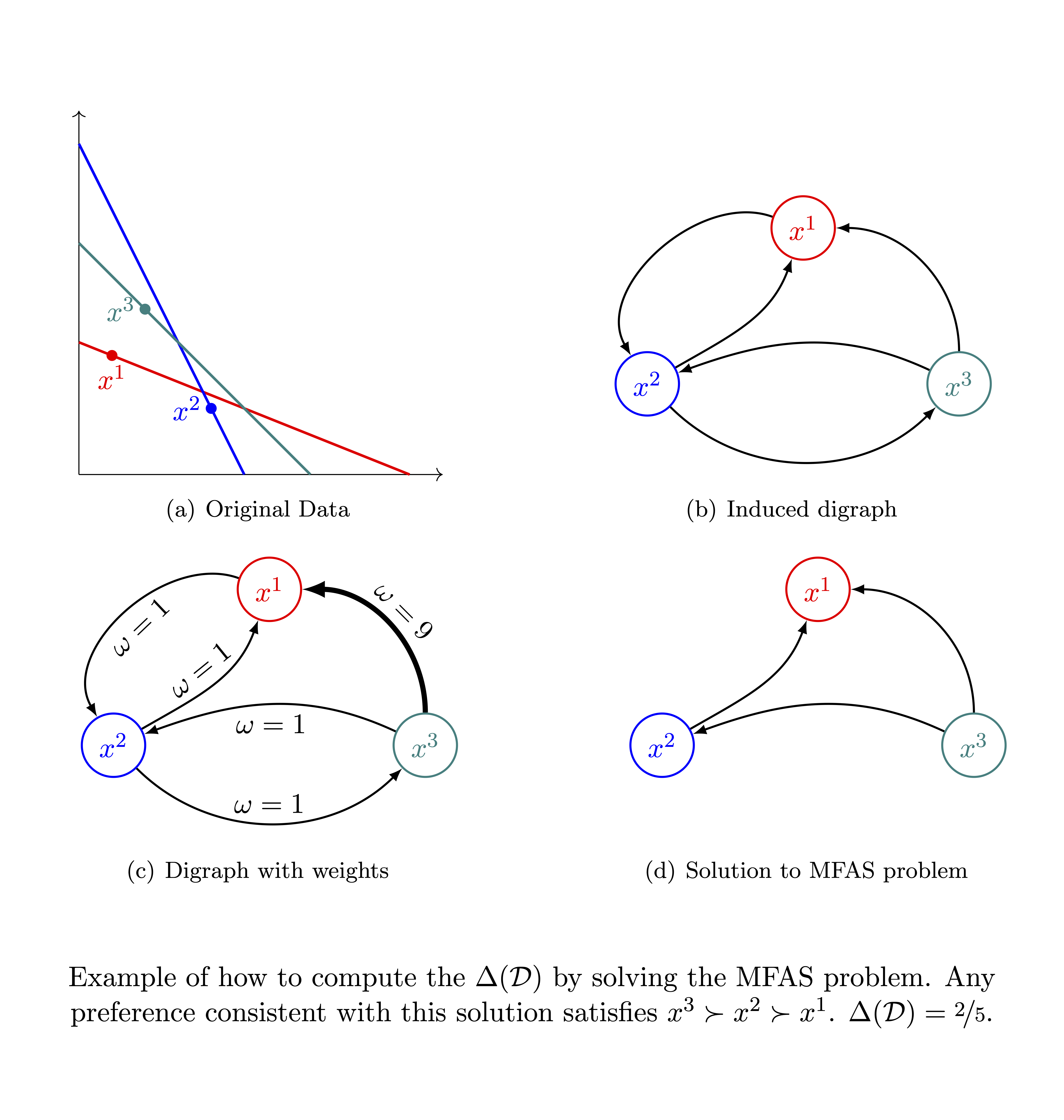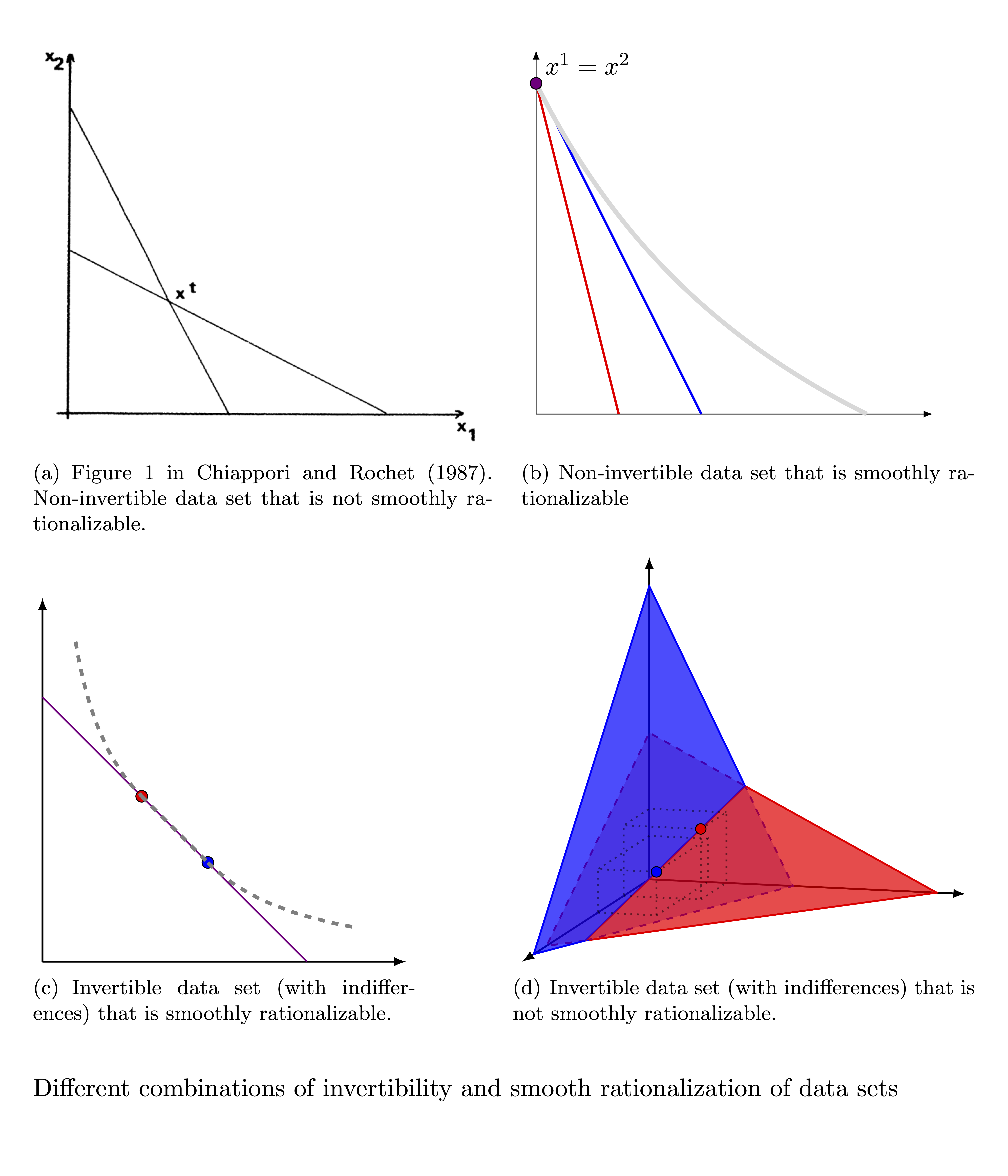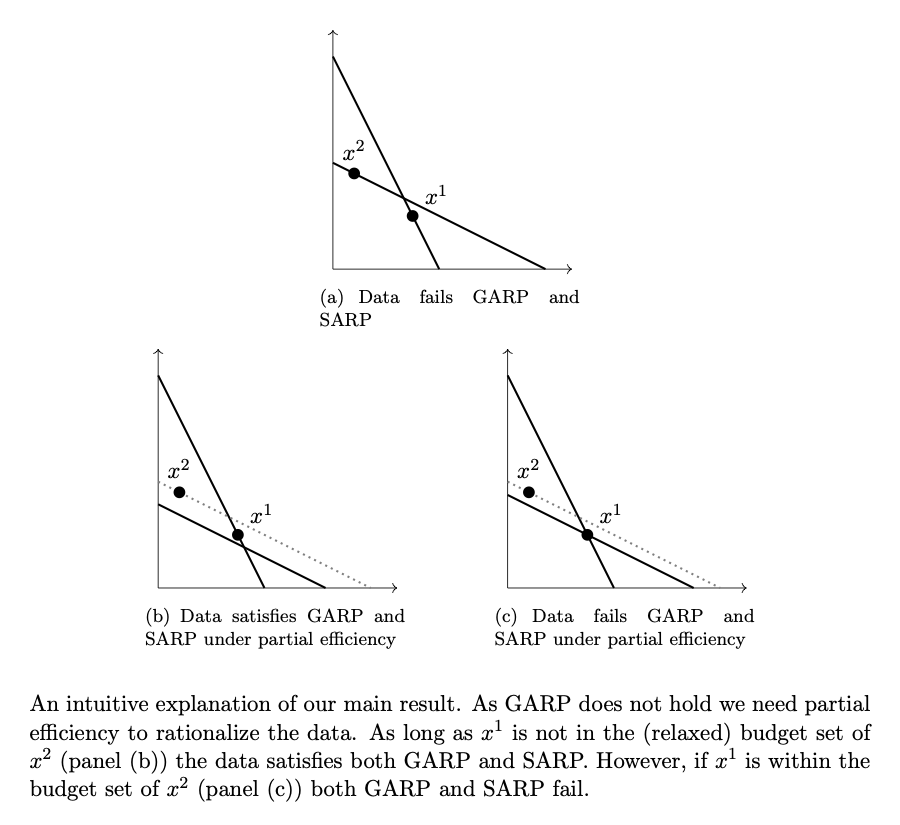Welcome! I am a Postdoctoral Scholar in the Department of Economics at the University of California, Berkeley. I have broad interests in microeconomic theory and methodological questions in economics. My research focuses on revealed preference theory.

My email is cugarte@berkeley.edu

## Working Papers#### Preference Recoverability from Inconsistent Choices

We study the analysis of choices imperfectly aligned with the preference relation that drives them. First, we develop a measure of decision-making quality that, unlike the existing ones, ensures to asymptotically measure the distance between the subject's choices and her underlying preference (instead of some preference). We then use such a measure to propose a statistically consistent preference estimator. Empirical results suggest consistency is a relevant property when recovering preferences, especially for complex choice environments, compared to estimators based on intuitive motivations.#### Smooth Rationalization

Economic models usually endow agents with (well-behaved) differentiable utilities. However, the behavioral implications of such an assumption are unclear. We study conditions under which choices can be rationalized by a differentiable utility, i.e., smoothly rationalized. Differentiability implies that indifferent choices have the same marginal rate of substitution. Starting from this observation, we develop an exact test for smooth rationalization. We also show that the existence of higher-order derivatives, commonly used for comparative statics, is empirically costless. We test smooth rationalization into several experimental data sets and find that, in most cases, choices are consistent with a differentiable utility.#### The generality of the Strong Axiom

Economic research usually endows consumers with a single-valued demand function. When choices are rationalizable, this assumption can be tested by the Strong Axiom of Revealed Preferences, SARP, as if they fail such a test, the demand is set-valued. We extend this test to non-rationalizable choices using partial efficiency, the most popular method to recover preferences. Under partial efficiency, a single-valued demand cannot be tested; furthermore, it can always be chosen to be infinitely differentiable. Hence, the existence of a single-valued, infinitely differentiable demand is falsified if, and only if, choices are rationalizable but fail SARP, which we do not observe in laboratory data. From an empirical standpoint, our results suggest that assuming a differentiable demand does not carry a cost.